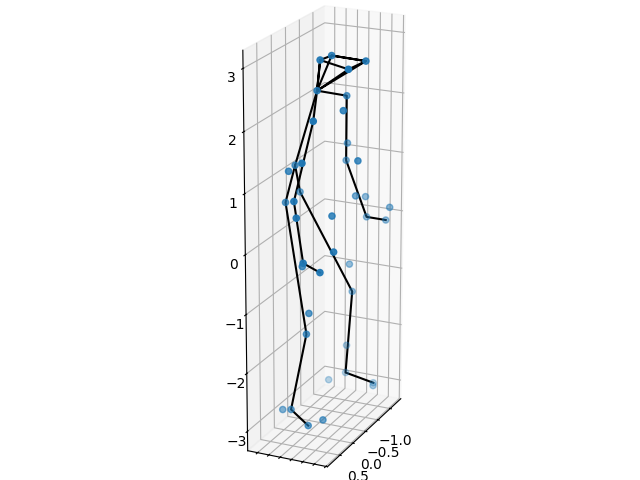# Non-rigid structure-from-motion (NRSfM)#

In computer vision, structure-from-motion (SfM) is an imaging technique for estimating three-dimensional structures from two-dimensional images. Theoretically, the problem is generally well-posed when considering rigid objects, meaning that the objects do not move or deform in the scene. However, non-static scenes are still relevant and have gained increased popularity among researchers in recent years. This is known as non-rigid structure-from-motion.

In this tutorial, we will consider motion capture (MOCAP). This is a special case, where we use images from multiple camera views to compute the 3D positions of specifically designed markers that track the motion of a person (or object) performing various tasks.

## Non-rigid shapes#

To make the problem well-posed, one has to control the complexity of the deformations using some minor assumptions on the possible space of object shapes. This is not a weird thing to do: consider e.g. the human body, we have different joints that bend and turn in a finite amount of ways; the skeleton itself is rigid and not capable of such deformations. For this reason, Bregler et al. 1 suggested that all movements (or shapes) can be represented by a low-dimensional basis. In the context of motion capture, this means that every movement a person does can be considered a combination of core movements (or basis shapes).

Mathematically speaking, this translates to any motion being a linear combination of the basis shapes, i.e. assuming there are $$K$$ basis shapes, any non-rigid shape $$X_i$$ can be written as

$X_i = \sum_{i=1}^K c_{ik}B_k$

where $$c_{ik}$$ are the basis coefficients and $$B_k$$ are the basis shapes. Here, $$X_i$$ is a $$3\times N$$ matrix where each column is a point in 3D space.

## The CMU MOCAP dataset#

Let us first try to understand the data we are given. We will use the Pickup instance from the CMU MOCAP dataset, which depicts a person picking something up from the floor.

import matplotlib.pyplot as plt
import matplotlib.animation as animation
import numpy as np
import scipy as sp

plt.close('all')
np.random.seed(0)
X_gt = data['X_gt']
markers = data['markers'].item()


First we view the first 3D poses. In order to easily visualize the person, we draw a skeleton between the markers corresponding to certain body parts. Note that these are not used in any other way.

def plot_first_3d_pose(ax, X, color='b', marker='o', linecolor='k'):
ax.scatter(X[0, :], X[1, :], X[2, :], color, marker=marker)
for j, ind in enumerate(markers.values()):
ax.plot(X[0, ind], X[1, ind], X[2, ind], '-', color=linecolor)
ax.set_box_aspect(np.ptp(X[:3, :], axis=1))
ax.view_init(20, 25)

fig = plt.figure()
plot_first_3d_pose(ax, X_gt)
plt.tight_layout()Now, we turn the attention to the data the algorithm is given, which is a sequence of 2D images from varying views. The goal is to recreate the 3D points, such as in the example above, from all timestamps.

M = data['M']
F = int(X_gt.shape / 3)

def _update(f: int):
X = M[2 * f:2 * f + 2, :]
lines.set_data(X[0, :], X[1, :])
for j, ind in enumerate(markers.values()):
lines[j + 1].set_data(X[0, ind], X[1, ind])
return lines

fig, ax = plt.subplots()
lines = ax.plot([], [], 'r.')
for _ in range(len(markers)):
lines.append(ax.plot([], [], 'k-'))
ax.set(xlim=(-2.5, 2.5), ylim=(-3.5, 3.5))
ax.set_aspect('equal')

ani = animation.FuncAnimation(fig, _update, F, interval=25, blit=True)